# Olympiad Test: Pattern - 1

Test Description

## 15 Questions MCQ Test Science Olympiad Class 7 | Olympiad Test: Pattern - 1

Olympiad Test: Pattern - 1 for Class 7 2023 is part of Science Olympiad Class 7 preparation. The Olympiad Test: Pattern - 1 questions and answers have been prepared according to the Class 7 exam syllabus.The Olympiad Test: Pattern - 1 MCQs are made for Class 7 2023 Exam. Find important definitions, questions, notes, meanings, examples, exercises, MCQs and online tests for Olympiad Test: Pattern - 1 below.
 1 Crore+ students have signed up on EduRev. Have you?
Olympiad Test: Pattern - 1 - Question 1

### ​Directions: Choose the appropriate number which follow the given pattern.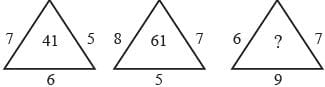Detailed Solution for Olympiad Test: Pattern - 1 - Question 1

7 × 5 + 6 = 41
8 × 7 + 5 = 61
6 × 7 + 9 = 51

Olympiad Test: Pattern - 1 - Question 2

### ​​Directions: Choose the appropriate number which follow the given pattern.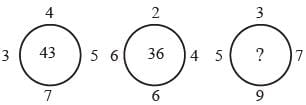Detailed Solution for Olympiad Test: Pattern - 1 - Question 2

7 × 4 + 3 × 5 = 28 + 15 = 43
6 × 2 + 6 × 4 = 12 + 24 = 36
9 × 3 + 5 × 7 = 27 + 35 = 62

Olympiad Test: Pattern - 1 - Question 3

### ​​Directions: Choose the appropriate number which follow the given pattern.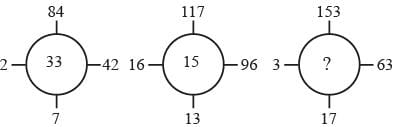Detailed Solution for Olympiad Test: Pattern - 1 - Question 3

84 ÷ 7 + 42 ÷ 2 = 12 + 21 = 33
117 ÷ 13 + 96 ÷ 16 = 9 + 6 = 15
153 ÷ 17 + 63 ÷ 3 = 9 + 21 = 30

Olympiad Test: Pattern - 1 - Question 4

Directions: Choose the appropriate number which follow the given pattern.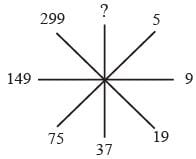Detailed Solution for Olympiad Test: Pattern - 1 - Question 4

5 × 2 – 1 = 9
9 × 2 + 1 = 19
19 × 2 – 1 = 37
37 × 2 + 1 = 75
75 × 2 – 1 = 149
149 × 2 + 1 = 299
299 × 2 – 1 = 597

Olympiad Test: Pattern - 1 - Question 5

​​​​Directions: Choose the appropriate number which follow the given pattern.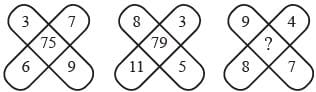Detailed Solution for Olympiad Test: Pattern - 1 - Question 5

3 × 7 + 6 × 9 = 21 + 54 = 75
8 × 3 + 11 × 5 = 24 + 55 = 79
9 × 4 + 8 × 7 = 36 + 56 = 92

Olympiad Test: Pattern - 1 - Question 6

​​​​Directions: Choose the appropriate number which follow the given pattern.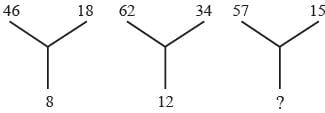Detailed Solution for Olympiad Test: Pattern - 1 - Question 6

(46 + 18) ÷ 8 = 64 ÷ 8 = 8
(62 + 34) ÷ 8 = 96 ÷ 8 = 12
(57 + 15) ÷ 8 = 72 ÷ 8 = 9

Olympiad Test: Pattern - 1 - Question 7

​​​​​Directions: Choose the appropriate number which follow the given pattern.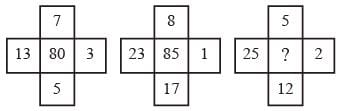Detailed Solution for Olympiad Test: Pattern - 1 - Question 7

(13 – 5)2 + (7 – 3)2 = 64 + 16 = 80
(23 – 17)2 + (8 – 1)2 = 36 + 49 = 85
(25 – 12)2 + (5 – 2)2 = 169 + 9 = 178

Olympiad Test: Pattern - 1 - Question 8

​​​​​Directions: Choose the appropriate number which follow the given pattern.​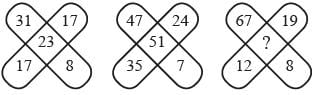Detailed Solution for Olympiad Test: Pattern - 1 - Question 8

(31 + 17) – (17 + 8) = 48 – 25 = 23
(47 + 35) – (24 + 7) = 82 – 31 = 51
(67 + 12) – (19 + 8) = 79 – 27 = 52

Olympiad Test: Pattern - 1 - Question 9

​​​​​Directions: Choose the appropriate number which follow the given pattern.​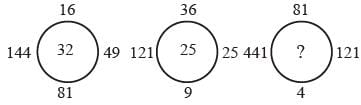Detailed Solution for Olympiad Test: Pattern - 1 - Question 9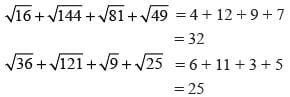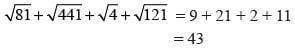Olympiad Test: Pattern - 1 - Question 10

​​​​​​Directions: Choose the appropriate number which follow the given pattern.​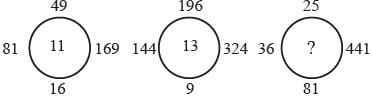Detailed Solution for Olympiad Test: Pattern - 1 - Question 10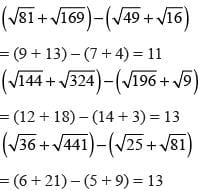Olympiad Test: Pattern - 1 - Question 11

​​​​​​Directions: Choose the appropriate number which follow the given pattern.​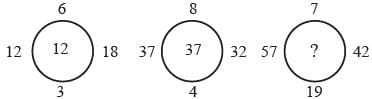Detailed Solution for Olympiad Test: Pattern - 1 - Question 11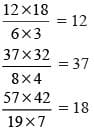Olympiad Test: Pattern - 1 - Question 12

​​​​​​Directions: Choose the appropriate number which follow the given pattern.​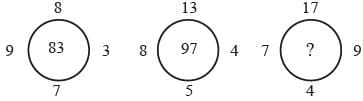Detailed Solution for Olympiad Test: Pattern - 1 - Question 12

(8 × 7) + (9 × 3) = 56 + 27 = 83
(13 × 5) + (8 × 4) = 65 + 32 = 97
(17 × 4) + (7 × 9) = 68 + 63 = 131

Olympiad Test: Pattern - 1 - Question 13

​​​​​​Directions: Choose the appropriate number which follow the given pattern.​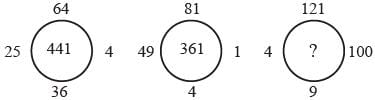Detailed Solution for Olympiad Test: Pattern - 1 - Question 13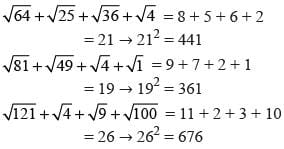Olympiad Test: Pattern - 1 - Question 14

​​​​​​Directions: Choose the appropriate number which follow the given pattern.​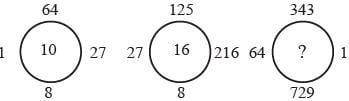Detailed Solution for Olympiad Test: Pattern - 1 - Question 14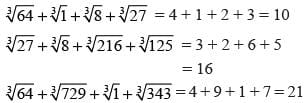Olympiad Test: Pattern - 1 - Question 15

​​​​​​Directions: Choose the appropriate number which follow the given pattern.​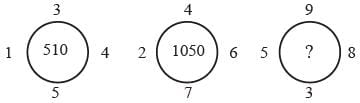Detailed Solution for Olympiad Test: Pattern - 1 - Question 15

(12 + 52 + 42 + 32) × 10 = 510
(22 + 72 + 62 + 42) × 10 = 1050
(52 + 32 + 82 + 92)  × 10 = 1790(Scan QR code)